# Tensor Product - Knapp - Theorem 6.10 ... Further Question

Gold Member
I am reading Anthony W. Knapp's book: Basic Algebra in order to understand tensor products ... ...

I need some help with a further aspect of the proof of Theorem 6.10 in Section 6 of Chapter VI: Multilinear Algebra ...

The text of Theorem 6.10 reads as follows: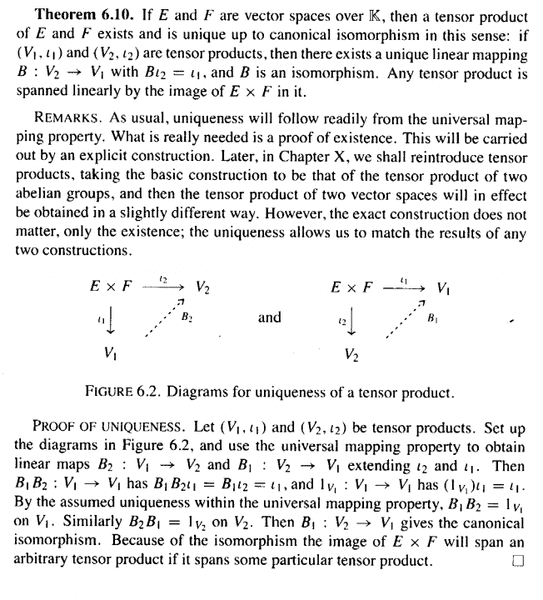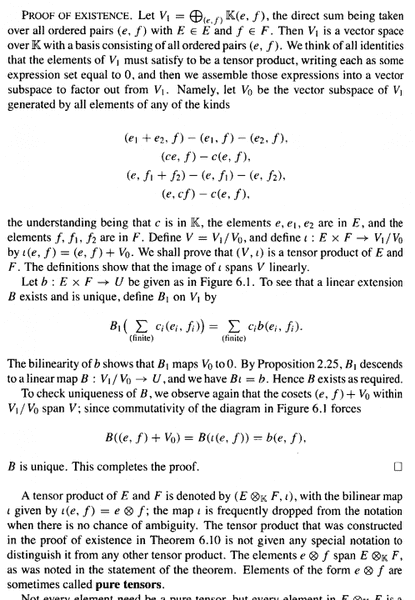The above proof mentions Figure 6.1 which is provided below ... as follows: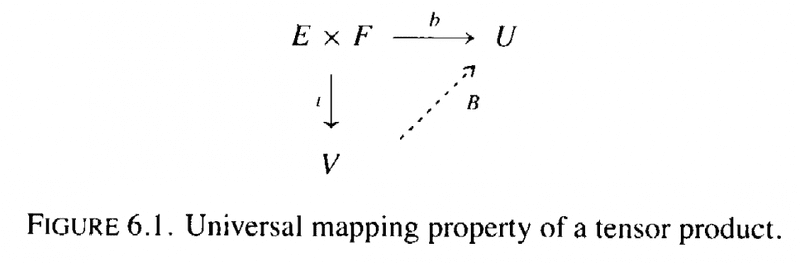In the above text, in the proof of Theorem 6.10 under "PROOF OF EXISTENCE" we read:

" ... ... The bilinearity of $b$ shows that $B_1$ maps $V_0$ to $0$. By Proposition 2.25, $B_1$ descends to a linear map $B \ : \ V_1/V_0 \longrightarrow U$, and we have $Bi = b$. "

My questions are as follows:

Question 1

Can someone please give a detailed demonstration of how the bilinearity of $b$ shows that $B_1$ maps $V_0$ to $0$?

Question 2

Can someone please explain what is meant by "$B_1$ descends to a linear map $B \ : \ V_1/V_0 \longrightarrow U$" and show why this is the case ... also showing why/how $Bi = b$ ... ... ?

Hope someone can help ...

Peter

===========================================================

*** EDIT ***

The above post mentions Proposition 2.25 so I am providing the text ... as follows: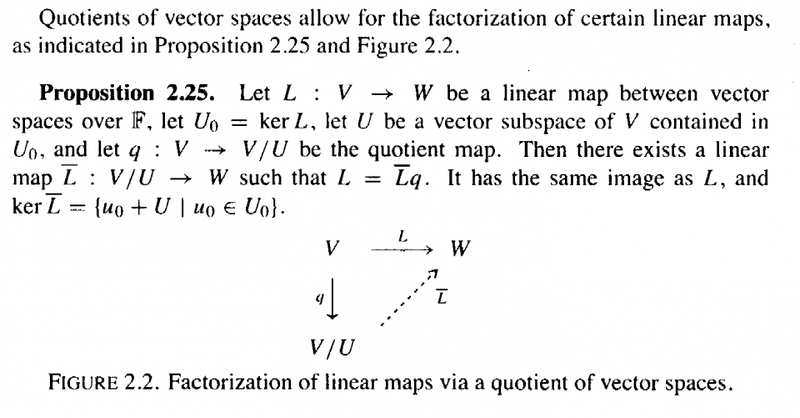============================================================

*** EDIT 2 ***

After a little reflection it appears that the answer to my Question 2 above should "fall out" or result from matching the situation in Theorem 6.10 to that in Proposition 2.25 ... also I have noticed a remark of Knapp's following the statement of Proposition 2.25 which reads as follows: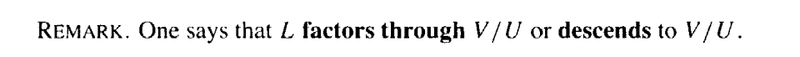So that explains the language: "$B_1$ descends to a linear map $B \ : \ V_1/V_0 \longrightarrow U$" ... ...

BUT ... I remain perplexed over question 1 ...

Peter

Last edited:

## Answers and Replies

Gold Member
I am reading Anthony W. Knapp's book: Basic Algebra in order to understand tensor products ... ...

I need some help with a further aspect of the proof of Theorem 6.10 in Section 6 of Chapter VI: Multilinear Algebra ...

The text of Theorem 6.10 reads as follows: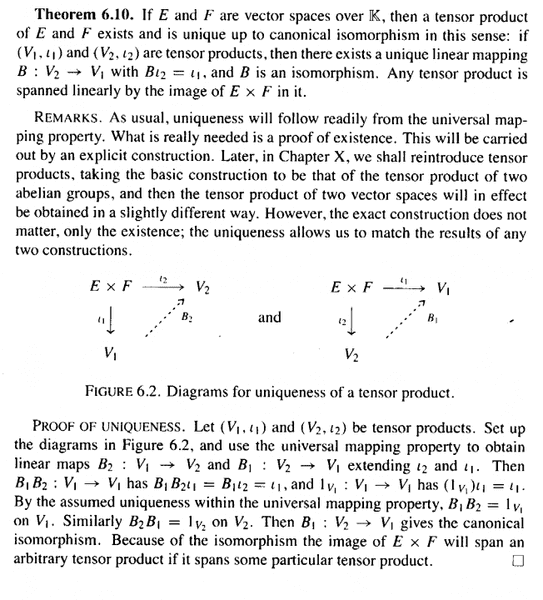The above proof mentions Figure 6.1 which is provided below ... as follows: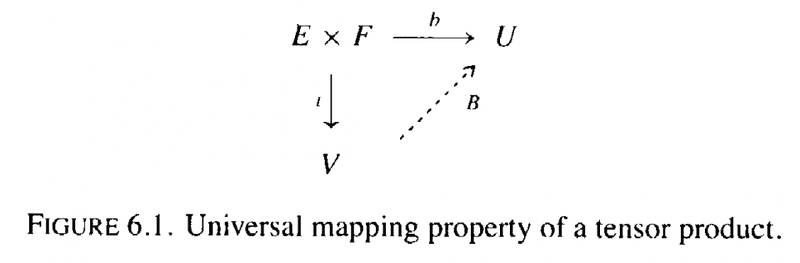In the above text, in the proof of Theorem 6.10 under "PROOF OF EXISTENCE" we read:

" ... ... The bilinearity of $b$ shows that $B_1$ maps $V_0$ to $0$. By Proposition 2.25, $B_1$ descends to a linear map $B \ : \ V_1/V_0 \longrightarrow U$, and we have $Bi = b$. "

My questions are as follows:

Question 1

Can someone please give a detailed demonstration of how the bilinearity of $b$ shows that $B_1$ maps $V_0$ to $0$?

Question 2

Can someone please explain what is meant by "$B_1$ descends to a linear map $B \ : \ V_1/V_0 \longrightarrow U$" and show why this is the case ... also showing why/how $Bi = b$ ... ... ?

Hope someone can help ...

Peter

===========================================================

*** EDIT ***

The above post mentions Proposition 2.25 so I am providing the text ... as follows: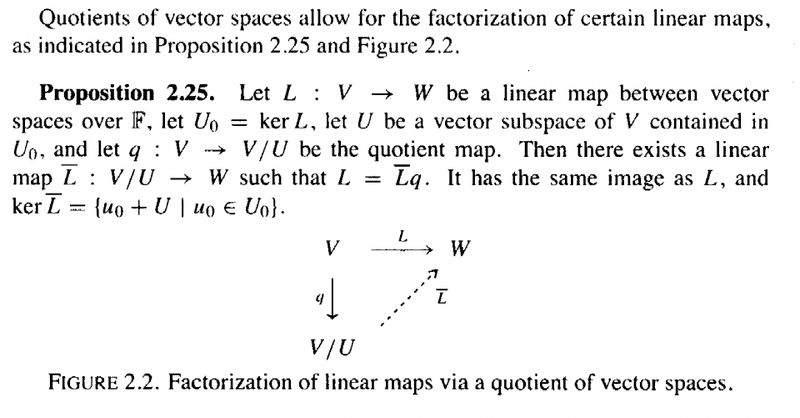============================================================

*** EDIT 2 ***

After a little reflection it appears that the answer to my Question 2 above should "fall out" or result from matching the situation in Theorem 6.10 to that in Proposition 2.25 ... also I have noticed a remark of Knapp's following the statement of Proposition 2.25 which reads as follows:So that explains the language: "$B_1$ descends to a linear map $B \ : \ V_1/V_0 \longrightarrow U$" ... ...

BUT ... I remain perplexed over question 1 ...

Peter

... ... BUT NOTE ... after further reflection and work ...

I am having trouble applying Proposition 2.25 to Theorem 6.10 ... SO ... Question 2 remains a problem ... hope someone can help ...

AND ... I remain perplexed over question 1 ...

Peter

lavinia
Science Advisor
Gold Member
Here is a way of thinking that may help with tensor products.

When one multiplies two numbers ##xy## ,for instance real or complex numbers, then the rules of ordinary multiplication and addition say that

##(ax)y = a(xy) = x(ay)##, ##(x_1 + x_2)y = x_1y + x_2y## and ##x(y_1 + y_2) = xy_1 + xy_2##

One would like to have exactly the same rules apply when ##x## and ##y## are vectors in two(usually different) vector spaces and ##a## is a scalar. Since it makes no sense to multiply vectors one needs to decide what a multiplication would mean. Your book defines the tensor product as the solution to a universal mapping problem. andrewkirk defines it in another way.

Whatever the construction one gets "products" ##x⊗y## in a new vector space, ##V⊗W##, that satisfy the rules of multiplication and addition. That is:

* ##a(x⊗y) = (ax)⊗y = x⊗(ay)##, ##(x_1+x_2)⊗y = x_1⊗y + x_2⊗y## and ##x⊗(y_1+y_2) = x⊗y_1+x⊗y_2##

This new vector space will be all linear combinations ##Σ_{i}a_{i}x_{i}⊗y_{i}## subject to the required rules of multiplication and addition. That is: the tensor product is just the vectors space of all symbols ##Σ_{i}a_{i}x_{i}⊗y_{i}## subject to the equivalence relations *

One can think of the tensor product of two vector spaces completely formally in this way. You tell yourself, " However it was constructed, this is what is must look like."

It is easy to check that a linear map from ##V⊗W## into another vector space,##U##, determines a bilinear map from ##V##x##W## into ##U## and visa versa.

Here is an exercise: Let ##V## be a vector space over the real numbers and view the complex numbers ##C## as a vectors space over the real numbers as well. What is the space ##V⊗C##? Is it a complex vector space?

Last edited:
•Math Amateur
lavinia
Science Advisor
Gold Member
Inner products can also be defined on free abelian groups. ##g(x,y)## is a symmetric positive definite bilinear form that takes values in the integers. There is the additional condition that for every linear map ##L## of ##V## into the integers there is a unique ##v_0## such that
##L(w) = g(w,v_0)## for all ##w## in ##L##.

- Show that there is only one inner product on the integers.

Last edited: